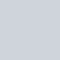# 題目出處

47. Permutations II

medium

# 個人範例程式碼

``````class Solution:
def permuteUnique(self, nums: List[int]) -> List[List[int]]:
if not nums:
return nums

nums.sort()
self.ans = []
self.dfs(nums, [])
return self.ans

def dfs(self, nums, permutations):
# end of recursion
if not nums:
self.ans.append(list(permutations))
return

# define and split
for i, num in enumerate(nums):
if i > 0 and nums[i] == nums[i-1]: # if duplicate, we only record first time
continue

permutations.append(num)
self.dfs(nums[:i] + nums[i+1:], permutations)
permutations.pop() # backtracking
``````

## 算法說明

• 此題目的前一題，差別在這題需要多處理重複的元素，可參考：

【Leetcode】python –  Permutations 個人解法筆記 #重要題型

### 處理重複

``````nums.sort()
``````

``````if i > 0 and nums[i] == nums[i-1]: # if duplicate, we only record first time
continue
``````

# Reference